## Deep Learning with Keras and TensorFlow

Certification Training
8494 Learners
7 Chapters +

# Training Deep Neural Nets

Welcome to the sixth lesson, ‘Training Deep Neural Nets’ of the Deep Learning Tutorial, which is a part of the Deep Learning (with TensorFlow) Certification Course offered by Simplilearn. This lesson gives you an overview of how to train Deep Neural Nets along regularization techniques to reduce overfitting.

Let us begin with the objectives of this lesson.

## Objectives

After completing this lesson on Training Deep Neural Nets, you’ll be able to:

• Discuss solutions to speed up neural networks

• Explain regularization techniques to reduce overfitting

## Developing Faster Neural Networks

Let us discuss how to develop faster neural networks in the following sections.

## Problems in Deep Neural Networks

Assume a neural net of 10 layers, each containing hundreds of neurons connected by hundreds of thousands of connections. The network is likely to cause the following problems:

• It results in vanishing gradients (or exploding gradients) that make lower layers very hard to train.

• It leads to slow training

• A model with millions of parameters would overfit the training set.

Gradients often get smaller and smaller as the algorithm progresses down to the lower layers.

As a result, the Gradient Descent update leaves the lower layer connection weights virtually unchanged, and training never converges to a good solution. This is called vanishing gradients problem.

Weight adjustment is contingent on minimizing the cost function using gradient descent. So, if the gradients dilute (or explode), the gradient descent process fails to arrive at a global minimum for loss. Without loss minimization, the model cannot be trained.

## Gradients in a Sigmoid Activation Function

The vanishing gradient problem is visible in the sigmoid graph, the most common activation function used.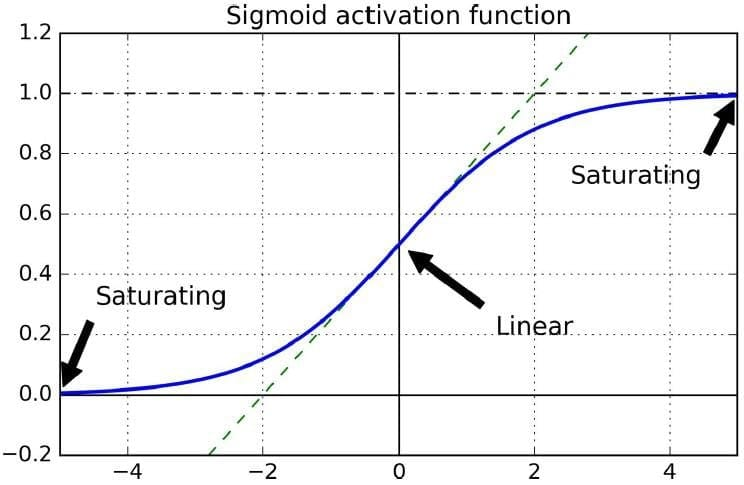When the input is highly positive or negative, the response is close to 0 or 1 with little change. Derivative in these areas is very close to 0. So, during backpropagation (from right to left in a neural net), the gradient gets smaller and smaller toward lower layers, with little left of it finally.

## How to Develop Faster Neural Nets?

The following techniques help solve vanishing / exploding gradient problem, as well as speed up the learning process:

• Applying a good initialization strategy for the connection weights (example: Xavier Initialization)

• Using a good activation function (example: ReLU activation)

• Using batch normalization

• Reusing parts of a pre-trained network

• Using a faster optimizer

• Increasing the learning rate

## The Solution for a Faster Neural Network

For faster Neural Network, the solutions are discussed as follows:

### Applying a good initialization strategy for the connection weights - Xavier Initialization

Researchers Glorot and Bengio have argued that for the signal to flow properly (forward and backward in a neural net) without vanishing/exploding gradients, the variance of the outputs of each layer needs to be equal to the variance of inputs. Also, the gradients should have equal variance before and after flowing through a layer in the reverse direction.

If we assume that ninputs and noutputs refer to the number of input and output connections for the layer whose weights are being initialized, the weights must be initialized randomly as described below (when using logistic activation function):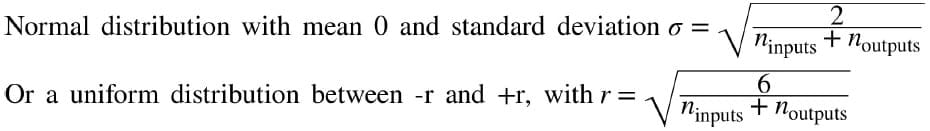This is called Xavier initialization or Glorot initialization.

### Using a good activation function - ReLU Activation Function

ReLU activation function is a non-saturating function (doesn’t saturate for positive values).

No saturation implies that the gradients do not dilute to zero, and one can use gradient descent to adjust weights in order to achieve convergence.

It provides faster convergence than Sigmoid function.

### Leaky ReLu Activation Function

ReLU suffers from dying ReLU problem: during training, the output of many neurons starts becoming 0 (caused by dead neurons). This happens even faster if one uses a large learning rate.

A variant of ReLU called a leaky ReLU solves this problem. This is defined as:

LeakyReLUα(z) = max(αz,z)

The hyperparameter α defines how much the function “leaks.” It is the slope of the function for z < 0 and is typically set to 0.01. This small slope ensures that leaky ReLUs never die.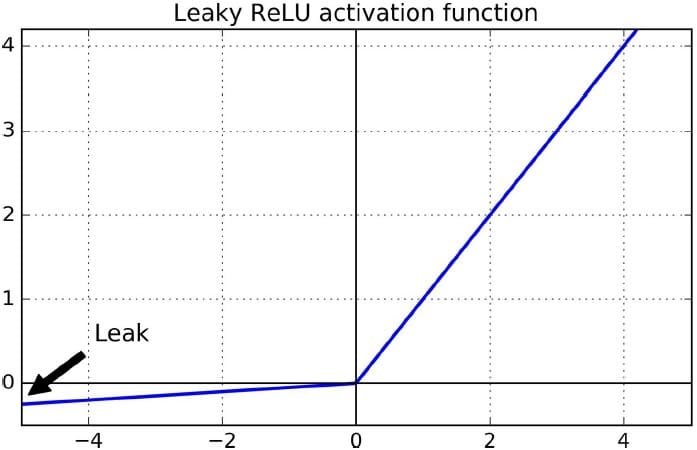### ELU Activation Function

The exponential linear unit (ELU) was proposed in 2015 and outperformed all the ReLU variants in experiments.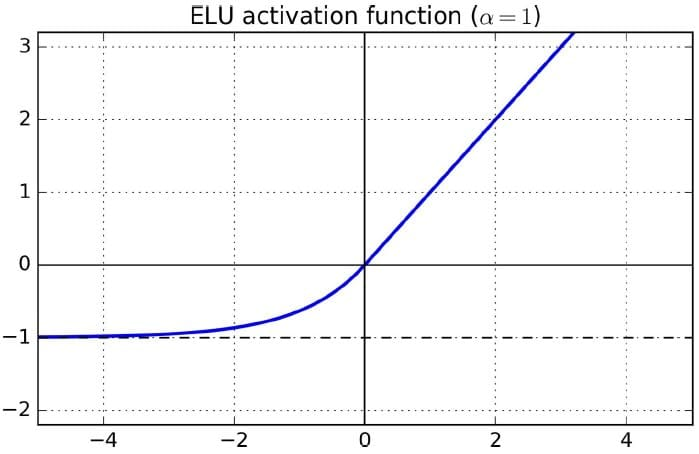Training time was reduced.

Neural network performed better on the test set.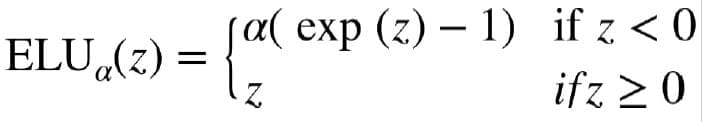### ELU vs. ReLU

ELU is preferred over ReLU as:

• It takes on negative values when z < 0, which allows the unit to have an average output closer to 0. This helps alleviate the vanishing gradients problem.

• It has a nonzero gradient for z < 0, which avoids the dying units issue.

• The function is smooth everywhere, including around z = 0. This helps speed up Gradient Descent as it does not bounce as much left and right of z = 0.

### Defining Leaky ReLu And ELU In Tensorflow

Leaky ReLU in Tensorflow:

def leaky_relu(z, name=None):

return tf.maximum(0.01 * z, z, name=name)

hidden1 = fully-connected(X, n-hidden1, activation_fn=leaky_relu)

ELU in Tensorflow:

hidden1 = fully-connected(X, n-hidden1, activation_fn=tf.nn.elu)

In general: ELU > leaky ReLU (and its variants) > ReLU > tanh > logistic"

### Using Batch Normalization

In a 2015 paper, Sergey Ioffe and Christian Szegedy proposed a technique called Batch Normalization (BN) to address the vanishing/exploding gradients problems.

The technique consists of adding an operation in the model just before the activation function of each layer, zero-centering, and normalizing the inputs. It then involves scaling and shifting the result using two new parameters per layer (one for scaling, the other for shifting).

In other words, this operation lets the model learn the optimal scale and mean of the inputs for each layer.

The advantages of using Batch Normalization are:

• Works with saturating activation functions like tanh or logistic

• Networks are much less sensitive to weight initialization

• Allows using larger learning rates, speeding up training

• Acts like a regularizer, reducing the need for other regularization techniques

### Reusing parts of a pre-trained network - Transfer Learning

Pre-trained neural nets can be used in current problems by replacing part of the neural net with layers specific to the problem at hand. This is called Transfer Learning.

### Transfer Learning: Using Pre-trained Layers

Transfer Learning involves using pre-trained layers from a prior trained neural network. This is a preferred practice for DNNs.

It speeds up training and requires less training data.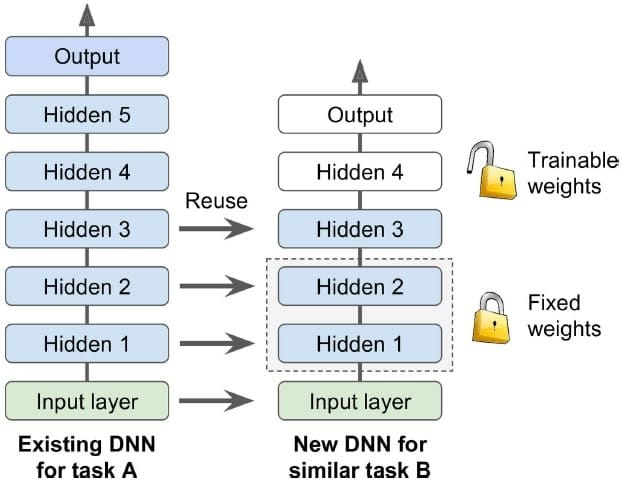Here, the weights of the first three hidden layers are said to be frozen (or fixed) when training the latter neural network.

If the input pictures of a new task don’t have the same size as an original task, add a preprocessing step to resize them to the size expected by the original model.

### Reusing A Tensorflow Model

To restore a pre-trained TensorFlow model and use it for the new task, use the following command:

[. . .]   # construct the original model

with tf.Session( ) as sess:

saver.restore(sess, “./my_original_model.ckpt”)

To restore selected parts of the original model only (say, the first three layers), use the following command: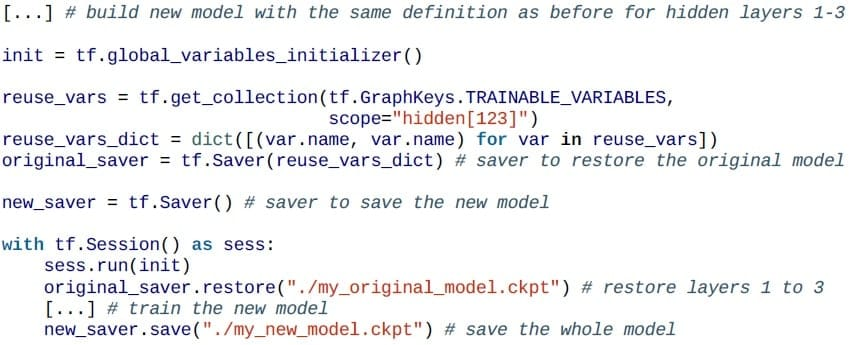The more similar the tasks are, the more layers you can reuse (starting with the lower layers). For very similar tasks, you can try keeping all the hidden layers and just replace the output layer.### Model Zoo

The community has published many pre-trained models that can be reused. This is called a model zoo.

TensorFlow has its own model zoo available at https://github.com/tensorflow/models. In particular, it contains most of the state-of-the-art image classification nets such as VGG, Inception, and ResNet(TensorFlow Slim package), including the code, the pre-trained models, and tools to download popular image datasets.

Another popular model zoo is Caffe’s Model Zoo. There is a converter available from Caffe to TensorFlow.

### Unsupervised pre-training

If there is a shortage of labeled training data and plenty of unlabeled data, the lower layers can be pre-trained using a feature detector mechanism like AutoEncoders or Restricted Boltzmann Machines (RBMs).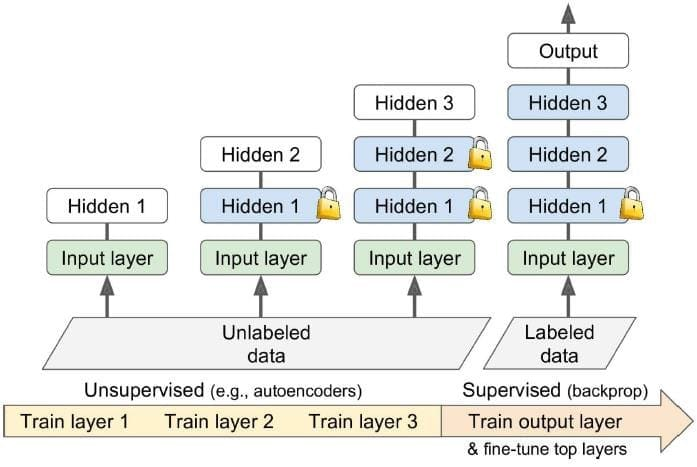Each layer is trained on the output of the previously trained layers (all layers except the one being trained are frozen). Once all layers have been trained this way, one can fine-tune the network using supervised learning (i.e., with backpropagation).

### Pre-training On An Auxiliary Task

If there is a shortage of training data of a certain type, pre-training on similar data can be done from other sources.

Example: For face detection of certain people, gathering hundreds of pictures per person may not be feasible.In this case, pre-training can be done on a broader set of random people from the internet.

Such a network will learn good feature detectors for faces. Parts of this pre-trained network can then be reused to train a model on a new set of face images.

Training with unlabeled data

In order to pre-train layers for language modeling, one could follow this process:

• Mark the sentences as "good"

• Corrupt some of the sentences (include wrong grammar or usage) and mark these corrupted ones as "bad"

• Example: Consider a sentence “The dog sleeps.” Mark “The dog sleeps” as good. Mark “The dog they” as bad.

• A model trained to classify such good and bad sentences will learn about language, and its lower layers can be reused in different language tasks.

### Using a Faster Optimizer

The following optimizers (apart from the usual GradientDescentOptimizer) help speed up training:

• Momentum optimization

• RMSProp

AdamOptimizer is widely used as it offers great performance.

### Increasing the learning rate - Learning Rate Scheduler

High or low learning rates lead to problems.

The best solution is to start with a slightly higher learning rate and then reduce it gradually during training. There are some scheduling techniques to enable this.

Since AdaGrad, RMSProp, and Adam optimization automatically reduce the learning rate during training, it is not necessary to add an extra learning schedule.

## Regularization Techniques to Prevent Overfitting

Let us go through the regularization techniques used to prevent overfitting in the following sections.

## Regularization

Regularization is any modification made to the learning algorithm that reduces its generalization error but not its training error.

In addition to varying the set of functions or the set of features possible for training an algorithm to achieve optimal capacity, one can use other ways to achieve regularization.

## Cost Function

One such method is weight decay, which is added to the Cost function.

This approach not only minimizes the MSE (or mean-squared error) but also expresses the preference for the weights to have smaller squared L2 norm (i.e., smaller weights).

λ is a pre-set value. It influences the size of the weights allowed. A higher value of λ causes weights to be smaller in size (because minimizing the second term implies that if the pre-set value λ is large, smaller weights get learned, and vice versa)

This works well as smaller weights tend to cause less overfitting (of course, too small weights may cause underfitting).

Higher λ means lower weights and less overfitting.

Lower λ means higher weights and more overfitting.

## Regularizer

More generally, to regularize a model, a penalty is added to the Cost function. This is called a Regularizer: Ω(w)

Hence, the Cost function becomes:

J(w) = MSEtrain+ λ * Ω(w)

In case of weight decay, this penalty is represented by:

Ω(w) = wTw

In essence, in the weight decay example, linear functions with smaller weights were preferred. This was done by adding an extra term to minimize the Cost function.

## Avoiding Overfitting with Regularization

Regularization is a mechanism to introduce some kind of error in model training in order to prevent overfit to training data and create a more generalized model.

DNNs have tens of thousands of parameters, sometimes even millions. This can often lead to overfitting. Regularization techniques are used in preventing the overfitting issues:

• Early stopping

• ℓ1 and ℓ2 regularization

• Dropout

### Early Stopping

In early stopping, the training is interrupted when performance on the validation set starts dropping. In TensorFlow, this can be implemented with some limit on the number of training steps.

In practice, regularization is better when Early stopping is combined with other regularization techniques.

### ℓ1 And ℓ2 Regularization

Regularization is used to constrain the neural network’s weights (but typically not its biases). One way to do this in TensorFlow is to add appropriate regularization term to the loss function. This works for one hidden layer.

The code shows how you can add regularization loss (reg_losses) to the core loss function (base_loss).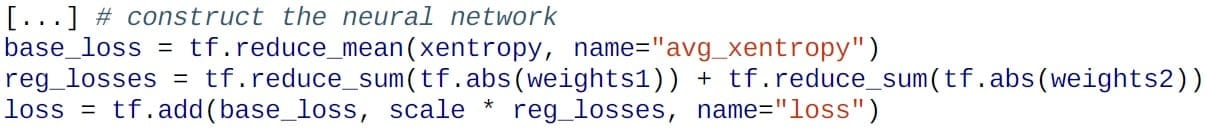For a higher number of layers, a more efficient way is to use the following command: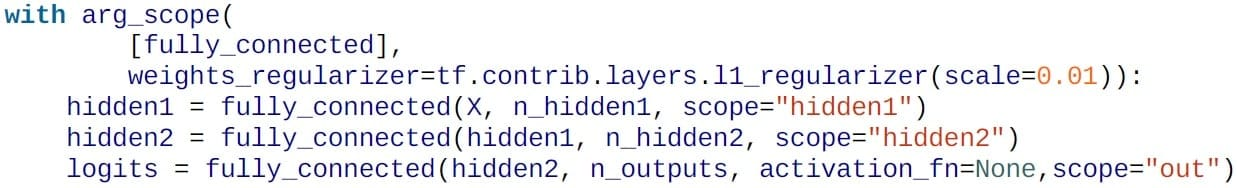The function l1_regularizer(), l2_regularizer(), or l1_l2_regularizer() can be used.

TensorFlow automatically adds the regularization nodes to a special collection containing all the regularization losses. Add regularization losses to the overall loss: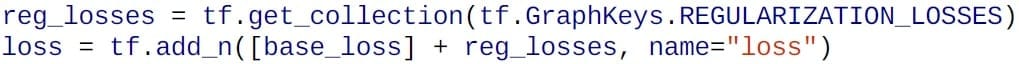### Dropout

Dropout is the idea of dropping certain neurons from calculations altogether in subsequent passes of the training loops.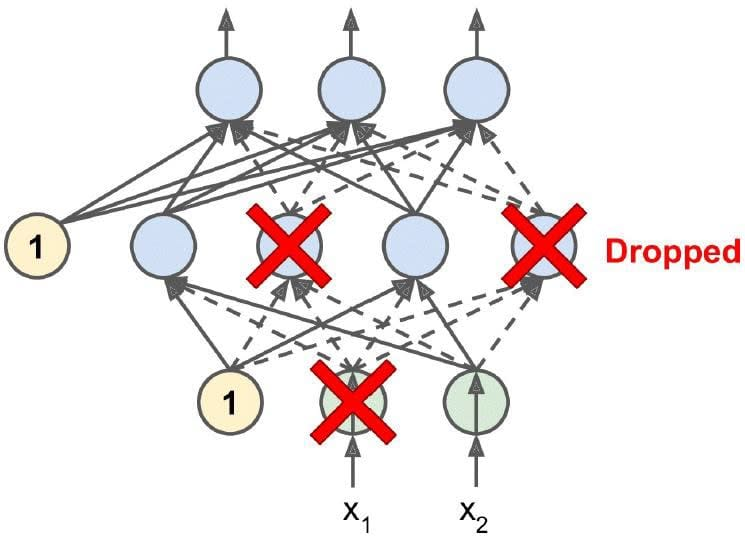This has the effect of adding inaccuracy in the weight adjustments for various neuron layers, hence causing less overfitting for the provided training data.

Dropout is the most popular method for regularization. It gives high accuracy rate. A 95% accurate network can reach 96-97% accuracy rate, which is considered a big jump.

In each training step, a few neurons from several of the layers (including input layer neurons or hidden layer neurons but not the output layer neurons) get dropped (not considered for subsequent processing). A neuron has a probability p of being dropped in a particular training step. This is called the dropout rate. Typically this value is set at p=50%.

### Why Dropout Works?

In each pass of the training loop, a neuron can be included or excluded. Hence, the surrounding neurons must become less sensitive to slight changes in inputs.

With each pass of the training loop, a new neural network is generated. In the end, we have a model trained on an ensemble of networks, which intuitively are able to generalize better.

During training, neurons are trained with approximately half the signal, whereas during testing or production, neurons will receive almost double the signal.

Hence, they are unlikely to perform well. So, one has to multiply connection weights with keep probability (1-p) after training OR during training and divide the output of neurons by the keep probability to mitigate this problem.

### Dropout In Tensorflow

The dropout function in TensorFlow randomly drops some items (setting them to 0) and divides the remaining items by the keep probability (during training only).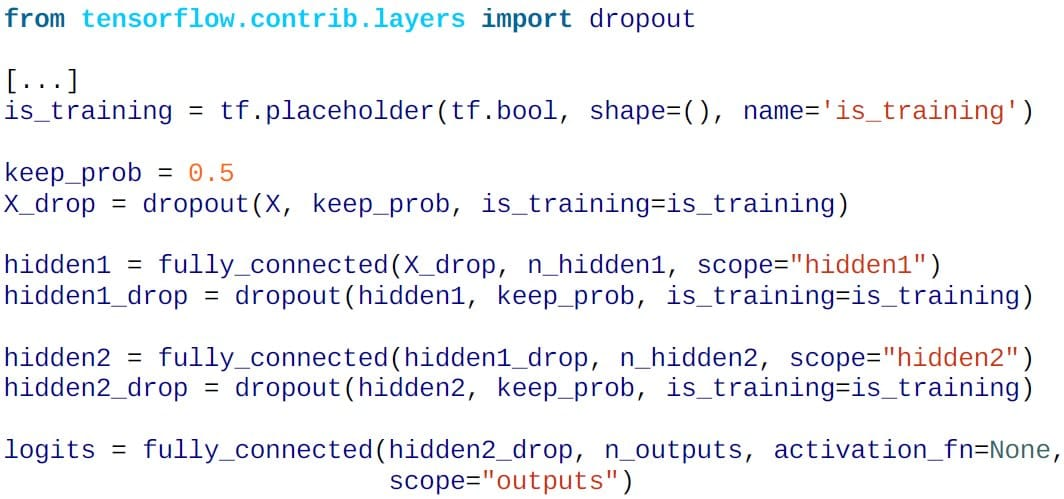## Default DNN Configuration

The table below shows the effective set of tuning criteria for achieving efficient and fast neural networks:

 Initialization He initialization Activation function ELU Normalization Batch Normalization Regularization Dropout Optimizer Adam Learning rate scheduler None

## Summary

Let us summarize what we have learned in this lesson:

• Deep Neural Nets face a host of issues like vanishing/exploding gradients, slow learning, and overfitting.

• Xavier initialization for weight initialization help solves vanishing/exploding gradients.

• ReLU, Leaky ReLU, and ELU are good activation functions for faster learning and non-saturating gradients.

• Batch normalization involves scaling and re-centering inputs to each layer. This helps in retaining gradients and faster learning.

• Transfer Learning involves using pre-trained layers from a different network to speed up learning.

• AdamOptimizer is one of the best optimizers to use for fast learning.

• Regularization techniques like early stopping, l1 or l2 regularization, and dropout help prevent overfitting.

## Conclusion

This concludes the lesson “Training Deep Neural Nets.” The next lesson talks about the topic “Introduction to Convolutional Neural Networks.

Related Courses
Learner Reviews
Related Articles
• Disclaimer
• PMP, PMI, PMBOK, CAPM, PgMP, PfMP, ACP, PBA, RMP, SP, and OPM3 are registered marks of the Project Management Institute, Inc.

Name*
Email*
Phone Number*